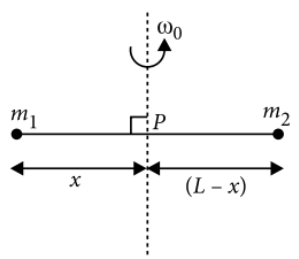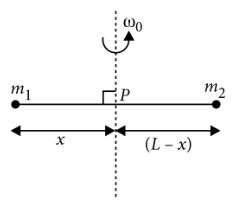Pure rotational motion and its kinetic energy
Question

# Point masses ${m}_{1}$  and ${m}_{2}$ are placed at the opposite ends of a rigid rod of length L, and negligible mass. The rod is to be set rotating about an axis perpendicular to it. The position of point P on this rod through which the axis should pass so that the work required to set the rod rotating with angular velocity ${\omega }_{0}$ is minimum, is given byDifficult
Solution

##Moment of inertia of the system about the axis of rotation (through point P ) is$I={m}_{1}{x}^{2}+{m}_{2}\left(L-x{\right)}^{2}$$W=\frac{1}{2}I{\omega }_{0}^{2}=\frac{1}{2}\left[{m}_{1}{x}^{2}+{m}_{2}\left(L-x{\right)}^{2}\right]{\omega }_{0}^{2}$

Get Instant Solutions